Creating a Sampling Distribution Simulation

Our goal is to simulate the collection of multiple samples. For this task, we are going to use the command randBin found under MATH → PROB → #7. The parameters are
randBin(n, p, repetitions) where n is the sample size, p is the proportion, and repetitions is the number of times you want to run the simulation.

For this example, the sample size is 40, the proportion is 0.3, and the number of simulation repetitions is 100. From the home screen, set randBin(40, 0.3, 100) and store the results in L1. randBin(40,0.3,100) → L1

Once L1is established, we need the proportion for each L1 sample in list L2. Type L1/40L2 on the home screen (or from the Lists in STAT #1, move up onto L2, and type L1/40 at the bottom of the screen, hit ENTER). You now have the Sampling Distribution of the Sample Proportions in L2. Using L2 you can find the sampling mean and the sampling standard deviation for the sample proportions.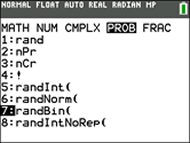MATH → PROB → #7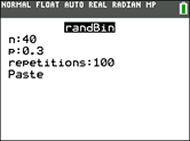n = sample size p = proportion repetitions = number of simulations neededStore the results in L1. It may take a few seconds for the generation of the values.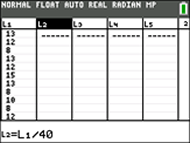In L2, store the proportions of the values in L1 divided by the sample size, 40.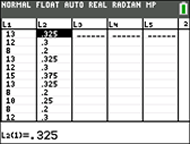The Sampling Distribution of Sample Proportions is now in L2.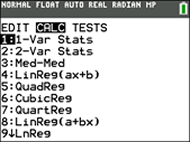STAT → CALC#1 Do a 1-Var Stat on L2.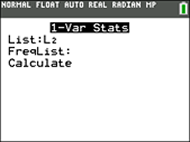Choose L2.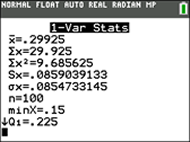The sampling mean and standard deviation are shown. The mean of all proportions is 0.29925.

To see this Sampling Distribution from L2 as a histogram, set STAT PLOT for histogram, ZOOM #9.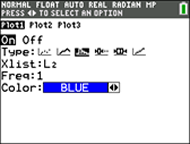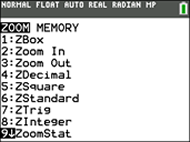Finding Your Way Around TABLE of  CONTENTS